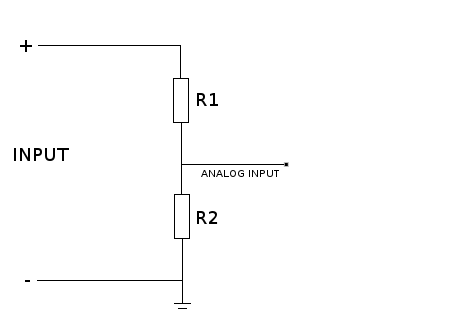# VoltMeter Release 0.0.0-alpha+sha.f83ce287d7

Voltmeter using a voltage divider

Keywords
voltmeter, arduino, voltage divider
MIT
Install
``` platformio lib install 1005 ```

# Arduino Voltmeter

This library uses a simple voltage divider for measure voltage. A voltage divider is very cheap and simple but very energy inefficient too so use it only for low currents.

## Using this library

This is a simple example for measuring voltages between 0 and 12 volts.

```#define INPUT_PIN A0
#define R1 15000
#define R2 10000
#define REFERENCE 4.8
VoltMeter voltMeter(INPUT_PIN, R1, R2, REFERENCE);
float voltage = voltMeter.getVoltage();```

Reference value is the maximum value your voltage divider should take. In this example, and input of 12V will produce 4.8V (because of this R1 and R2 combination). Its important that you know the maximum volage you are going to measure, otherwise you could burn your arduino pin. In this example if you connect it 20V, the output (the voltage recevied by the arduino pin) will be 8V and you will get a fried pin.

By default the REFERENCE value is 5, assuming that you adjust you R1 and R2 to get exactly 5V as ouput. Don't recommend that because resistors have tolerance so this value is not 100% exactly and you could damage your arduino pin or in the best case, you will take incorrect values. But for testing or getting aproximation values it is ok.

Of course you can use a combination of R1 and R2 to get 2.5V when the input is 12V (a security margin of 2.5V). Again, it depends on you what values are acceptable.

For build the voltage divider you can follow this schemaTo get your values for R1 and R2 just use an voltage divider calculator